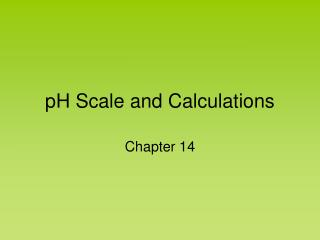# pH Scale and Calculations - PowerPoint PPT PresentationDownload PresentationpH Scale and Calculations

pH Scale and CalculationsDownload Presentation## pH Scale and Calculations

- - - - - - - - - - - - - - - - - - - - - - - - - - - E N D - - - - - - - - - - - - - - - - - - - - - - - - - - -
##### Presentation Transcript

1. pH Scale and Calculations Chapter 14

2. pH Scale • We use this scale to measure the strength of an acid or base. • pH is defined as the –log[H+] • pH can use the concentration of hydronium ions or hydrogen ions.

3. pH Scale 7 Acid Base 0 14 Zumdahl, Zumdahl, DeCoste, World of Chemistry2002, page 515

4. pH of Common Substances Timberlake, Chemistry 7th Edition, page 335

5. Ionization of Water Occasionally, in water, a H+ is transferred between H2O molecules . . . . . . . . H:O: + :O:H H:O:H + + :O:H- . . . . . . . . HH H water molecules hydronium hydroxide ion (+)ion (-) LecturePLUS Timberlake

6. Pure Water is Neutral Pure water contains small, but equal amounts of ions: H3O+ and OH- H2O + H2O H3O+ + OH- hydronium hydroxide ion ion 1 x 10-7 M1 x 10-7 M H3O+ OH-

7. Ion Product of Water Kw Kw = [ H3O+ ] [ OH- ] = [ 1 x 10-7 ][ 1 x 10-7 ] = 1 x 10-14

8. pH of Common Substance pH [H1+] [OH1-] pOH 14 1 x 10-14 1 x 10-0 0 13 1 x 10-13 1 x 10-1 1 12 1 x 10-12 1 x 10-2 2 11 1 x 10-11 1 x 10-3 3 10 1 x 10-10 1 x 10-4 4 9 1 x 10-9 1 x 10-5 5 8 1 x 10-8 1 x 10-6 6 6 1 x 10-6 1 x 10-8 8 5 1 x 10-5 1 x 10-9 9 4 1 x 10-4 1 x 10-10 10 3 1 x 10-3 1 x 10-11 11 2 1 x 10-2 1 x 10-12 12 1 1 x 10-1 1 x 10-13 13 0 1 x 100 1 x 10-14 14 NaOH, 0.1 M Household bleach Household ammonia Lime water Milk of magnesia Borax Baking soda Egg white, seawater Human blood, tears Milk Saliva Rain Black coffee Banana Tomatoes Wine Cola, vinegar Lemon juice Gastric juice More basic 7 1 x 10-7 1 x 10-7 7 More acidic

9. Acid – Base Concentrations 10-1 pH = 3 pH = 11 OH- H3O+ pH = 7 10-7 concentration (moles/L) H3O+ OH- OH- H3O+ 10-14 [H3O+]<[OH-] [H3O+]>[OH-] [H3O+]=[OH-] acidic solution neutral solution basic solution Timberlake, Chemistry 7th Edition, page 332

10. pH pH = -log [H+] Kelter, Carr, Scott, Chemistry A World of Choices 1999, page 285

11. Self-Ionization Of Water • Even the purest of water conducts electricity. This is due to the fact that water self-ionizes, that is, it creates a small amount of H3O+ and OH-. H2O + H2O º H3O+ + OH- Kw = [H3O+][OH-] • Kw - ion product of water Kw = 1.0 x 10-14 at 25 oC • This equilibrium constant is very important because it applies to all aqueous solutions - acids, bases, salts, and non-electrolytes - not just to pure water.

12. O H H H Self ionization reaction of water: + - O O + O + H H H H H

13. pH and pOH • pH = - log[H3O+] [H3O+] = 10-pH pOH = - log[OH-] [OH-] = 10-pOH • pKw = pH + pOH = 14.00 • neutral solution: [H3O+] = [OH-] = 10 –7 M pH = 7.0 acidic solution: [H3O+] > 10-7 M pH < 7.0 basic solution: [H3O+] < 10-7 M pH > 7.0

14. Learning Check pH A. The [H3O+] of tomato juice is 1 x 10-4 M. What is the pH of the solution? 1) - 4 2) 4 3) 8 B. The [OH-] of an ammonia solution is 1 x 10-3 M. What is the pH of the solution? 1) 3 2) 11 3) -11 LecturePLUS Timberlake

15. Solution pH4 A. pH = - log [ 1 x 10-4] = -(- 4) = 4 B. [H3O+] = 1 x 10-11 pH = - log [ 1 x 10- 11] = -(- 11) = 11 LecturePLUS Timberlake

16. Learning Check pH5 The pH of a soap is 10. What is the [H3O+] of the soap solution? 1) 1 x 10 - 4 M 2) 1 x 1010 M 3) 1 x 10 - 10 M LecturePLUS Timberlake

17. Solution pH5 The pH of a soap is 10. What is the [H3O+] of the soap solution? [H3O+] = 1 x 10-pH M = 1 x 10-10 M LecturePLUS Timberlake

18. pH on the Calculator [H3O+] is 4.5 x 10-6 M pH = -log(4.5 x10-6 ) = 5.35

19. Learning Check pH6 A soap solution has a [H3O+] = 2 x 10-8 M. What is the pH of the solution? 1) 8 2) 7.7 3) 6 LecturePLUS Timberlake

20. Solution pH6 A soap solution has a [H3O+] = 2.0 x 10-8 M. What is the pH of the solution? B) = 7.7

21. Calculating pH from pOH • The concentration of [OH–] in a cleaning product was found to be 10–3 mol L–1. • Find the pH of the solution.

22. Practise • (a) A 0.01 mol L–1 solution of HNO3 is prepared. Calculate the pH of the • solution. • (b) A 0.005 mol L–1 solution of H2SO4 is prepared. Assuming complete • ionisation, calculate the pH of the solution.

23. Sample Problem: Calculating the pH of a dilution • 50 mL of a solution of HCl of pH 2 is diluted so that the new pH is 5. What • volume of water was added?